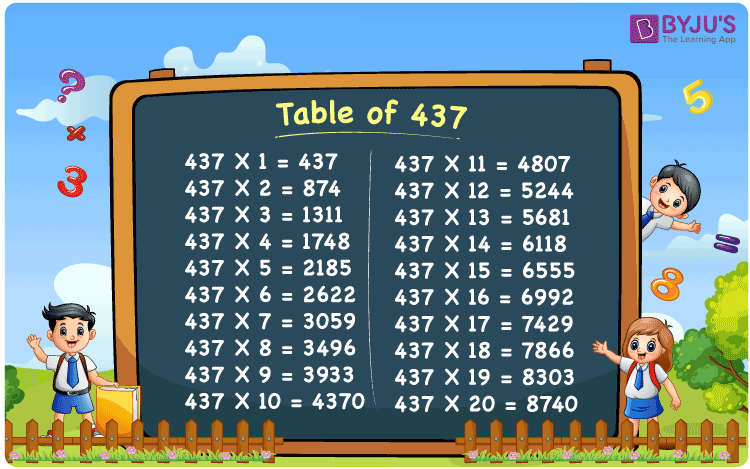Checkout JEE MAINS 2022 Question Paper Analysis : Checkout JEE MAINS 2022 Question Paper Analysis :

# Table of 437

The Table of 437 boosts problem-solving skills in students and candidates appearing for competitive exams. 437 times table is the multiplication table that involves one of the arithmetic operations known as multiplication. Students are suggested to memorise the multiplication tables, as it saves a lot of time while solving more problems. Get the PDF of the multiplication table of 437 from the link mentioned below.## What is the 437 Times Table?

The values gained by repeated addition of number 437 for n number of times is considered as the multiplication table of 437. Tables play a vital role in solving problems in a quick way. Refer to the multiplication table of 437 for the first 10 multiples from the below chart.

 437×1 = 437 437 437×2 = 874 437 + 437 = 874 437×3 = 1311 437 + 437 + 437 = 1311 437×4 = 1748 437 + 437 + 437 + 437 = 1748 437×5 = 2185 437 + 437 + 437 + 437 + 437 = 2185 437×6 = 2622 437 + 437 + 437 + 437 + 437 + 437 = 2622 437×7 = 3059 437 + 437 + 437 + 437 + 437 + 437 + 437 = 3059 437×8 = 3496 437 + 437 + 437 + 437 + 437 + 437 + 437 + 437 = 3496 437×9 = 3933 437 + 437 + 437 + 437 + 437 + 437 + 437 + 437 + 437 = 3933 437×10 = 4370 437 + 437 + 437 + 437 + 437 + 437 + 437 + 437 + 437 + 437 = 4370

## Multiplication Table of 437

Follow the chart mentioned below to learn the table of 437. The multiplication tables of 437 from 1 to 20 times are provided below.

 437 × 1 = 437 437 × 2 = 874 437 × 3 = 1311 437 × 4 = 1748 437 × 5 = 2185 437 × 6 = 2622 437 × 7 = 3059 437 × 8 = 3496 437 × 9 = 3933 437 × 10 = 4370 437 × 11 = 4807 437 × 12 = 5244 437 × 13 = 5681 437 × 14 = 6118 437 × 15 = 6555 437 × 16 = 6992 437 × 17 = 7429 437 × 18 = 7866 437 × 19 = 8303 437 × 20 = 8740

## Solved Example on the Table of 437

Example 1:

Calculate the product of all the prime factors of 437.

Solution:

The prime factors of 437 are 19, 23

Product of prime factors = 19 x 23 = 437

Hence, the required answer is 437.

## Frequently Asked Questions on Table of 437

### What is the sum of factors of 437?

Factors of 437 are 1, 19, 23, 437.
Sum of factors = 1 + 19 + 23 + 437 = 480.

### What is the value of 14 times 437?

14 times 437 is equal to 6118.

### Calculate 437 times 5 plus 15 minus 8.

437 times 5 plus 15 minus 8
= 437 x 15 + 15 – 8
= 6555 + 15 – 8
= 6562